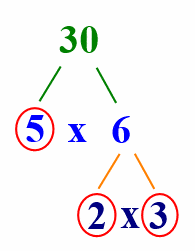# What is Prime Factorization?

All numbers are built from prime numbers. “Factors” are the numbers you multiply to get another number.

For example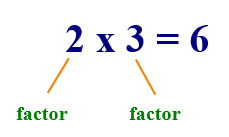“Prime factorization” is finding which prime numbers multiply to make the original number.

## What is a prime number?

And what is a prime number, you may ask?

Some number can only be divided by 1 or the number itself. These numbers are called prime numbers.

The prime numbers less than 30 are 2, 3, 5, 7, 11, 13, 17, 19, 23 and 29.

## Prime factorization using a factor tree

It’s easier to factor numbers to their prime factors with a factor tree. Here’s how it works. Say, we want to factor 24.First, 24 is factored into 4 x 6.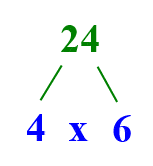However, neither 4 or 6 are prime numbers. So we need to continue factoring. 4 is factored into 2 x 2, and 6 is factored into 2 x 3.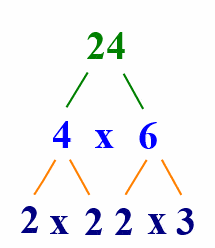2 and 3 are both prime numbers, so we don’t need to factor any further.

The prime factorization of 24 is 2 x 2 x 2 x 3.

Here’s another example, where the factorization looks a little different. Let’s find the prime factors of 30.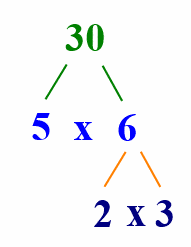The prime factorization of 30 is 5 x 2 x 3.

5 is a “leaf”. One way to easily identify them is to circle the prime factors.